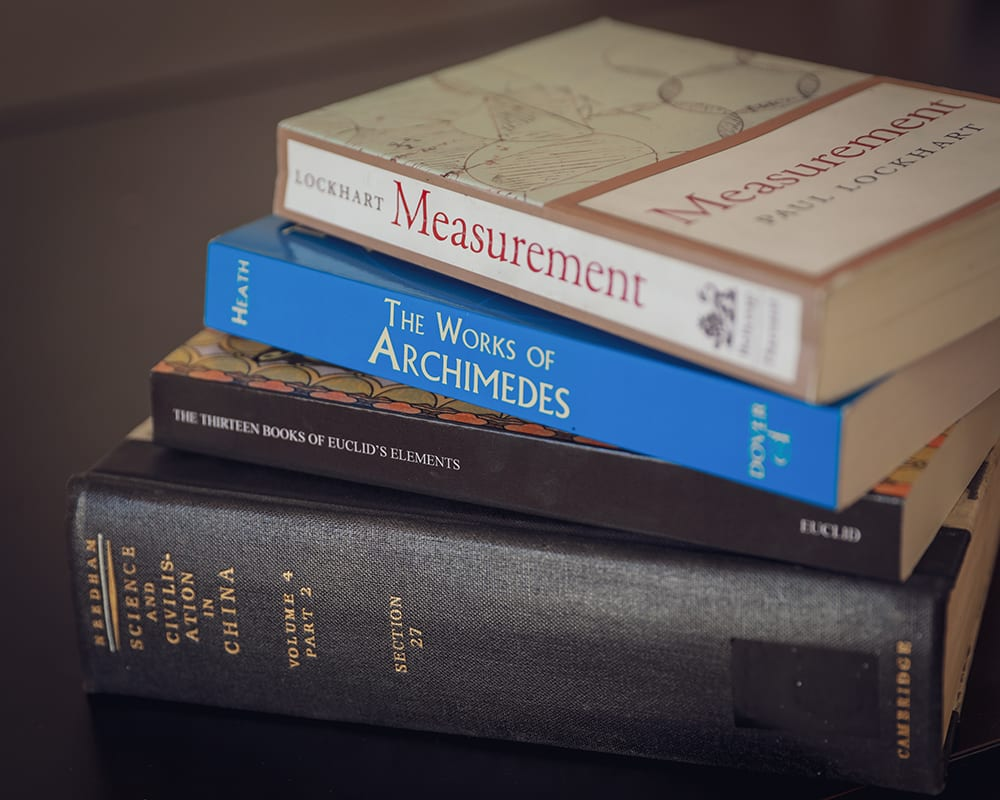High School Mathematics DepartmentMath comes from daily life. We encourage students to learn by investigation and become creative problem solvers in real-to-life mathematical scenarios. Classes in lower grade-levels incorporate foundational skills in algebra and strategic reasoning. Upper-grade levels explore advanced areas of math and their real-world applications.

The comprehensive program includes a range courses from Algebra I and Geometry to Calculus, Statistics, and Discrete Math. Students learn to develop independent thinking, connect visuals to algebraic representations, and become more confident with their ability to reason logically.

Course Description

• Algebra 1
• Geometry
• Algebra 2

Grades Offered: 9, 10

Prerequisites: 8th Grade Math or equivalent

UC Approved: Yes

In Algebra 1, students will write algebraic expressions, solve linear equations and simple linear systems, and graph relationships. They will solve quadratic equations by factoring, completing the square, and graphically. They will learn about rate of change, inequalities, monomial and polynomial expressions, exponents, and functions. By the end of the course, students will understand the practical applications and real-world uses of algebra.
*Students may take this course in 7th or 8th grade for high school credit.

Grades Offered: 9, 10

Prerequisites: Algebra 1 or equivalent

UC Approved: Yes

In Geometry 1, students will study a variety of topics, including geometric proofs and theorems, congruence, rotations and reflections, similarity, ratios, geometric properties of curves, and probability. Our math program takes a hands-on approach that gives students opportunities for kinetic learning, such as making their own geometric constructions using compasses and straightedge, to copy, reduce, and enlarge plane shapes, thus understanding similarity. They will also learn to write their own proofs and how to use geometric modeling.

Grades Offered: 9, 10

Prerequisites: Algebra 1 or equivalent

UC Approved: Yes

In Algebra 2, students will study many types of functions, including absolute-value, quadratic, polynomial, radical, exponential, logarithmic, and rational functions. They will expand their understanding of numbers to imaginary and complex numbers, solve different types of equations and inequalities, and learn to prove basic trigonometric identities. They will describe sequences and calculate series, study conics with emphasis on circles and parabolas, and expand their understanding of data analysis and statistics, using normal distribution.

• Pre-Calculus
• AP Calculus AB
• Discrete Math

Grades Offered: 10, 11, 12

Prerequisites: Algebra 2 or equivalent

UC Approved: Yes

In Precalculus, students will study the complex number plane, learn to simplify Polynomial, Rational, and Exponential Expressions, graph various functions showing key features, use trigonometric functions to model phenomena, such as sound, and systematically solve systems of linear equations and inequalities. They will also review functions from Algebra II, learn how to use vectors and matrices, how to describe inverse functions graphically and algebraically, and how to define functions using parameters and polar coordinates.

Grades Offered: 11, 12

Prerequisites: Algebra 2 and Pre-Calculus or equivalent

UC Approved: Yes

In AP Calculus, students will prepare for the AP test, learn how to calculate integrals, and use them to calculate areas and volumes. Students will learn the rules to calculate derivatives of functions and use them in various applications such as curve sketching and optimization problems, expand their understanding of differentiation and integration to exponential and logarithmic functions, and learn to solve differential equations using different methods.

Grades Offered: 11, 12

Prerequisites: Geometry and Algebra 2 or equivalent (Recommended: Pre-Calculus )

UC Approved: Yes

In Discrete Mathematics, students will be primarily solving mathematical problems; writing proofs and/or using mathematical induction; studying integers and their divisibility properties; analyzing, searching, and sorting algorithms; and working with graphs and sets. Other topics of the course include basic logic, lists and sets, relations and partitions, advanced proof techniques, recurrence relations, functions and their properties, permutations and symmetry, discrete probability theory, number theory, and graph theory.

Mathematics Department FacultyMs. Cornelia Ritter

Math Teacher
Ms. Ritter obtained a Masters degree in Physics from the University of Bern, Switzerland, in 1994, with a minor in mathematics. She received the teaching credentials for secondary schools in 1997 for mathematics and physics. She has more than 20 years of teaching experience. She left Switzerland in 2006, lived in Greece, India, and Malaysia, and came to the United States in 2010.Mr. Ray Chen

Science Teacher
Mr. Ray Chen received his Master of Science degree from Purdue University. He brings decades of work experience from global enterprises like Apple and Oracle, as well as years of experience from teaching previously at High School of the Arts and at other private schools.